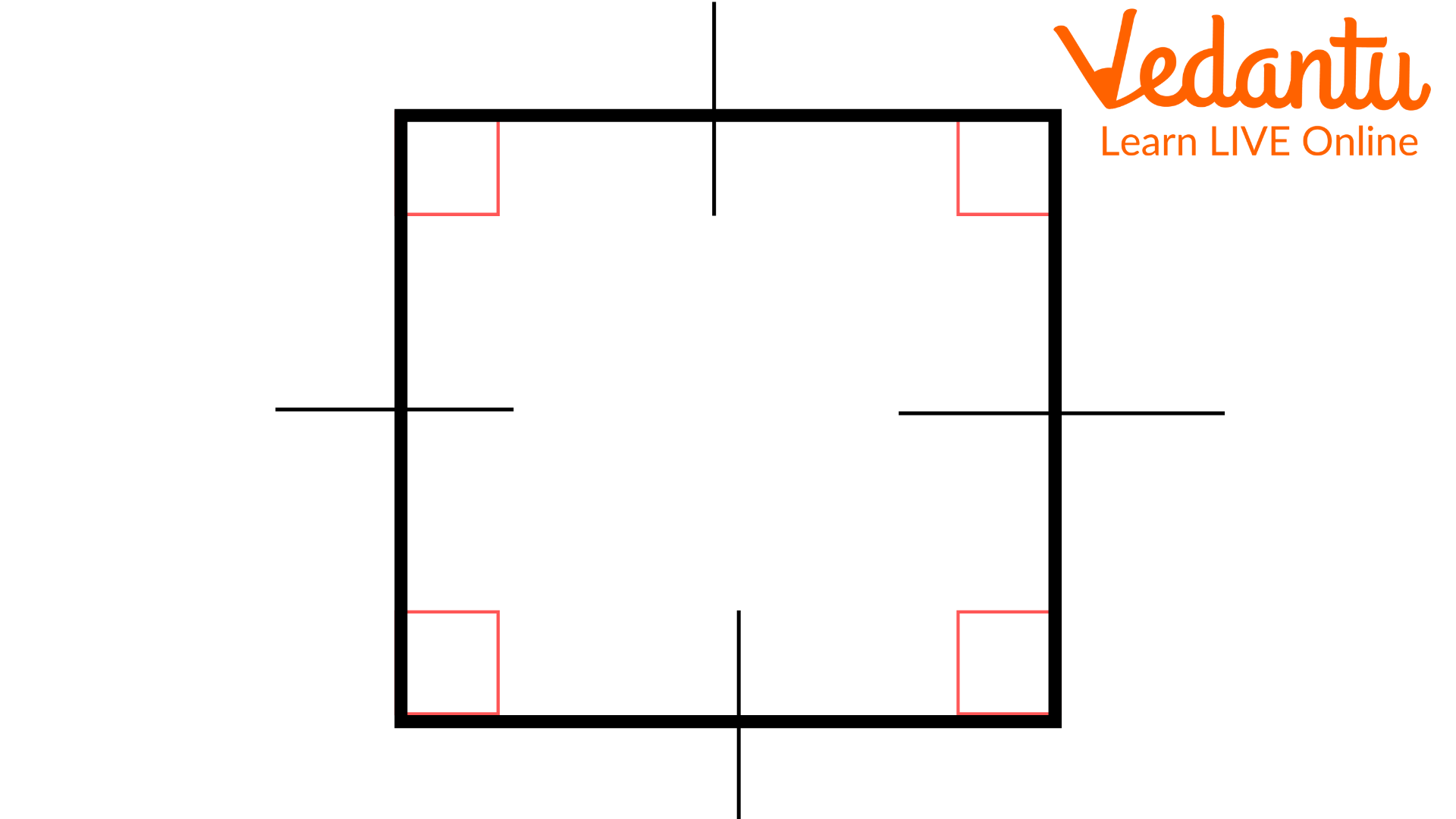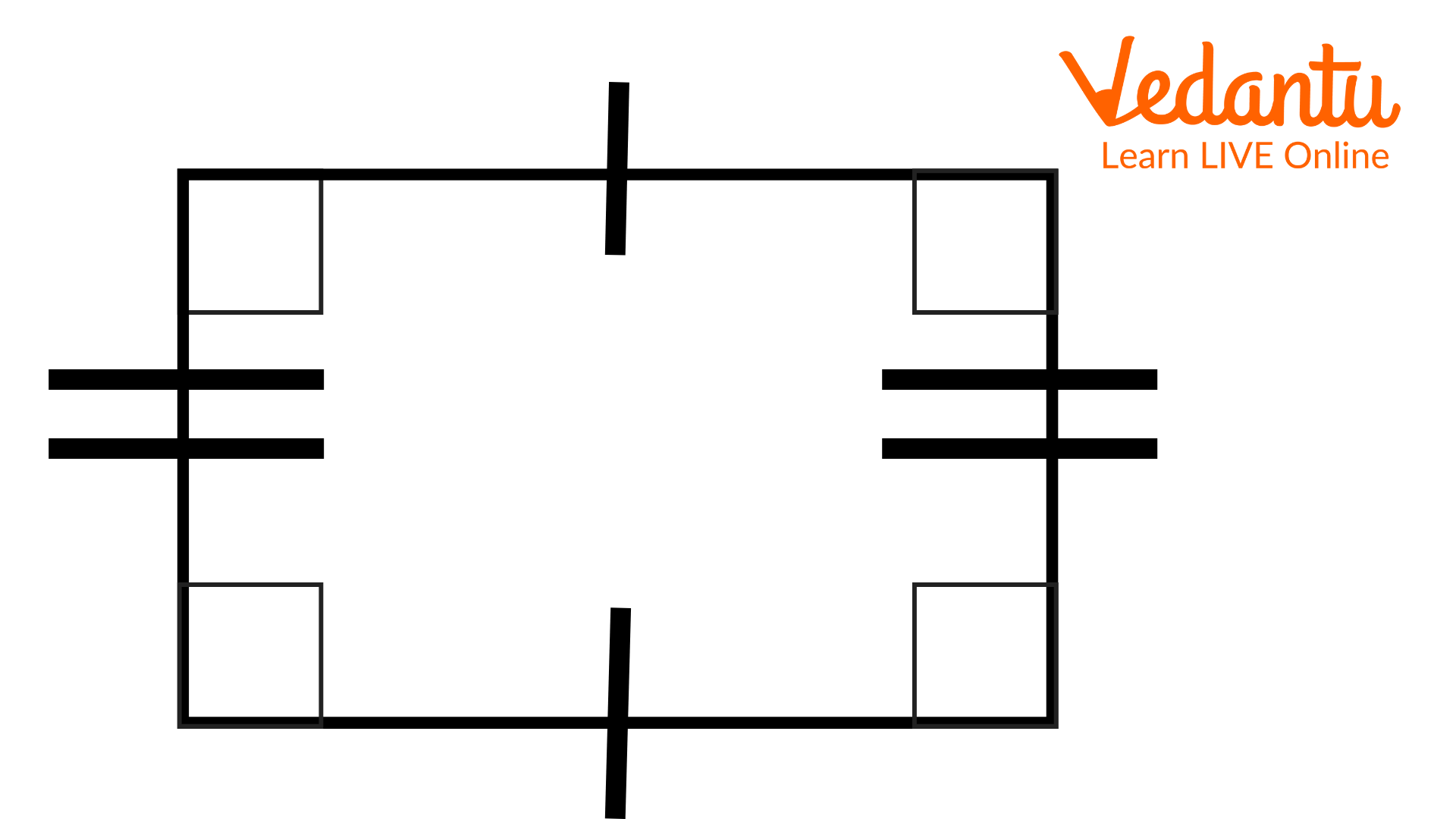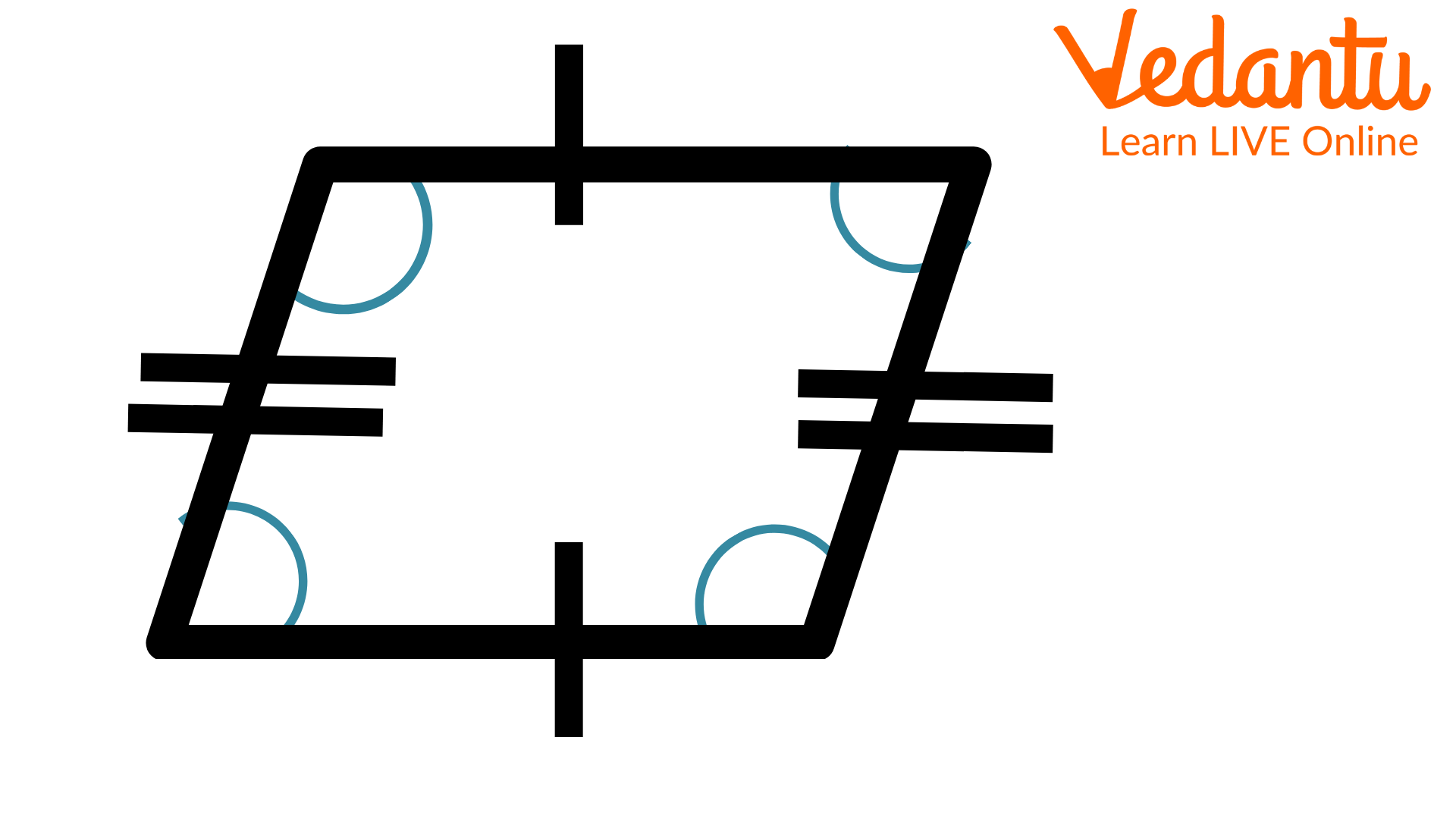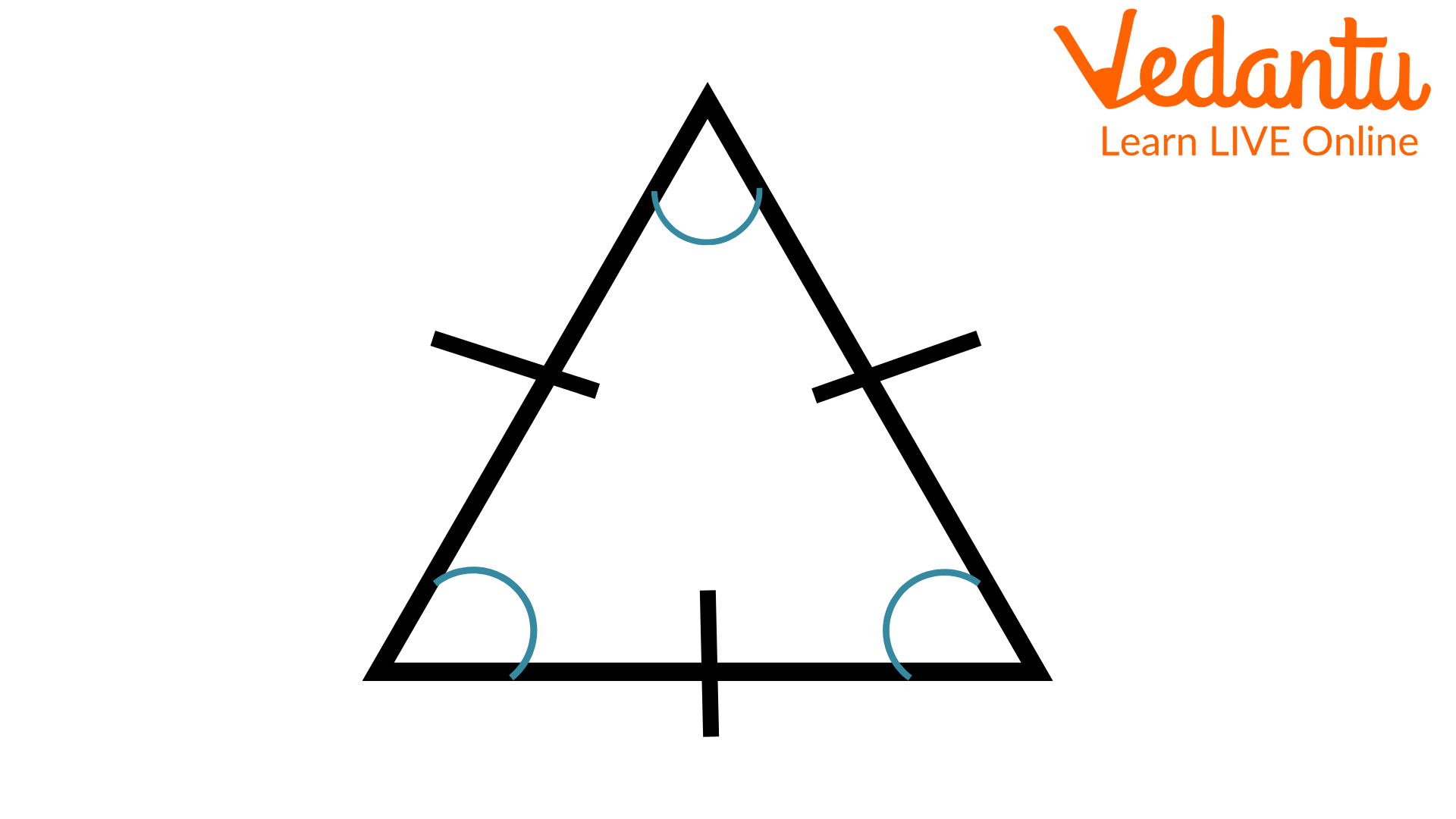Courses
Courses for Kids
Free study material
Offline Centres
More

# Shapes Having Sides of Equal LengthLast updated date: 25th Nov 2023
Total views: 150k
Views today: 3.50k## What are Sides of Equal Length?

Sides of equal length mean the sides of any object or shape are equal in length. We see many objects and shapes in our daily life that contain sides of equal length. Some geometrical shapes that contain equal lengths of sides are rectangle, square, isosceles triangle, parallelograms, rhombus, etc. Shapes can contain many numbers of sides of equal length such as hexagon, pentagon, etc. In this article, we will learn about the objects and geometrical shapes that contain sides of equal length.

## Geometric Shapes That Contain Sides of Equal Length

There are some shapes that contain sides of equal length. For example, your notebook is shaped in a manner that opposite sides are equal in length. Such a type of shape is called rectangular where opposite sides are of equal length. Let’s know about them in detail.

1. Square: All the sides are of equal length and all angles are 90° in measurement. The figure of a square is given below.A Square

1. Rectangle: In a rectangle, the opposite sides are of equal length. All the internal angles in a rectangle are 90°. The figure of a rectangle is given below.A Rectangle

1. Parallelogram: In a parallelogram, the opposite sides are equal in length as well as parallel. The opposite angles are equal in measurement. The figure of a parallelogram is given below.A parallelogram

1. Equilateral Triangle: In an equilateral triangle, all sides are of equal length and each angle is 60°. The figure of an equilateral triangle is given below.An Equilateral Triangle

## Conclusion

In the above article, some shapes are discussed with figures which contain sides of equal length. Some figures have all sides equal in length while some have opposite sides equal in length. Learn and remember the name of these shapes as they are important and can be seen in daily life. Try to identify them in the objects around you and measure and see if they have sides of equal length.

## FAQs on Shapes Having Sides of Equal Length

1. What shapes have all sides of the same length?

We have seen a lot of shapes and objects in our surroundings. Some of them contain equal length and some are not equal in length. There are some polygons that contain sides of equal lengths such as square, hexagon, pentagon etc. In a hexagon, all six sides are of equal length. If all sides of a triangle are equal in length, then it is called an equilateral triangle.

2. Which polygon has four sides of equal length?

Polygons are geometric shapes that are made up of at least three sides. Most of the common shapes that we see or study every day are polygons. For example, a wall is rectangular in shape where opposite sides are of equal length. There are some polygons where all four sides are equal in length such as square and rhombus. The difference between a square and a rhombus is that in a square, all the internal angles are 90o in measurement, and in a rhombus, it is not.